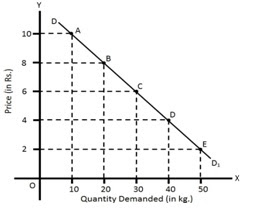# Law of Demand

Visits

## Law of Demand

It is one of the important laws of economics which was first propounded by neo-classical economist, Alfred Marshall. According to him, “Other things remaining the same, the amount demanded increases with a fall in price and diminishes with a rise in price.”

The law of demand states that all other things being equal, the quantity bought of a good or service is a function of price. As long as nothing else changes, people will buy less of something when its price rises. They'll buy more when its price falls. This means, there is inverse or opposite relationship between quantity demanded and price of a commodity.

This law is based on The Law of Diminishing Marginal utility. According to this law, when a person consumes more and more of a commodity, the utility from the latter units declines. Law of demand is based on the following assumptions;

• No change in the price of related commodities.
• No change in income of the consumer.
• No change in taste and preferences, customs, habit and fashion of the consumer.
• No change in the size of the population
• No expectation regarding the future change in price.

This law can be explained with the help of a demand schedule and demand curve as presented below:
 Price per kg Quantity Demanded Rs. 10 10 kg Rs. 8 20 kg Rs. 6 30 kg Rs. 4 40 kg Rs. 2 50 kg

The above demand schedule shows a negative relationship between price and quantity demanded a commodity.

Initially, when the price of a good is Rs.10 per kg, the quantity demanded by the consumer is 10 kg. As the price decrease from Rs.10 per kg to Rs.8 per kg and then to Rs.6 per kg, quantity demanded by the consumer increases from 10 kg to 20 kg and then to 30 kg respectively and so on. We can show, the above demand schedule through the following demand curve:In the above figure, price and quantity demanded are measured along the y-axis and x-axis respectively. By plotting various combinations of price and quantity demanded, we get a demand curve DD1 derived from points A, B, C, D and E. This is a downward sloping demand curve showing an inverse relationship between price and quantity demanded.